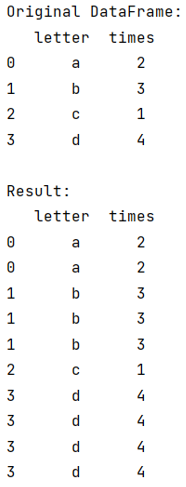# Repeat Rows in DataFrame N Times

Given a pandas dataframe, we have to repeat rows in dataframe n times.
Submitted by Pranit Sharma, on September 09, 2022

Pandas is a special tool that allows us to perform complex manipulations of data effectively and efficiently. Inside pandas, we mostly deal with a dataset in the form of DataFrame. DataFrames are 2-dimensional data structures in pandas. DataFrames consist of rows, columns, and data.

## Repeating rows in dataframe n times

Suppose, we have a DataFrame with two columns "letter" and "times", "times" is a numeric column and contains some integer values. We need to repeat the letter's value n number of times and n is its corresponding times value.

Rows in pandas are generally marked with the index number but in pandas, we can also assign index names according to the needs. In pandas, we can create, read, update, and delete a column or row value. The index is the number of rows that ranges from 0 to n-1, so if the index is 0, it represents the first row and the n-1th index represents the last row.

For this purpose, we will access the index of the DataFrame and for each index, we will use the repeat() method inside which we will pass the value of times column.

Let us understand with the help of an example,

## Python code to repeat rows in dataframe n times

```# Importing pandas package
import pandas as pd

# Creating a dictionary
d = {
'letter':['a','b','c','d'],
'times':[2,3,1,4]
}

# Creating DataFrame
df = pd.DataFrame(d)

# Display the DataFrame
print("Original DataFrame:\n",df,"\n")

# Repeating each row
result = df.loc[df.index.repeat(df.times)]

# Display result
print("Result:\n",result)
```

Output:What's New (MCQs)

Top Interview Coding Problems/Challenges!

Languages: » C » C++ » C++ STL » Java » Data Structure » C#.Net » Android » Kotlin » SQL
Web Technologies: » PHP » Python » JavaScript » CSS » Ajax » Node.js » Web programming/HTML
Solved programs: » C » C++ » DS » Java » C#
Aptitude que. & ans.: » C » C++ » Java » DBMS
Interview que. & ans.: » C » Embedded C » Java » SEO » HR
CS Subjects: » CS Basics » O.S. » Networks » DBMS » Embedded Systems » Cloud Computing
» Machine learning » CS Organizations » Linux » DOS
More: » Articles » Puzzles » News/Updates

© https://www.includehelp.com some rights reserved.